## 1. 基础概念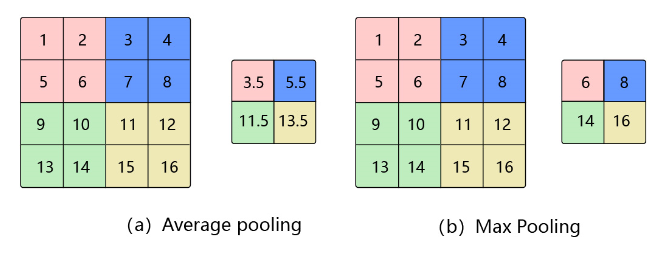### 1.3. K-max池化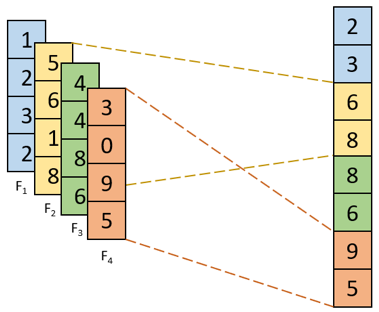## 2. 特点

 - 当输入数据做出少量平移时，经过池化后的大多数输出还能保持不变，因此，池化对微小的位置变化具有鲁棒性。例如 图3 中，输入矩阵向右平移一个像素值，使用最大池化后，结果与平移前依旧能保持不变。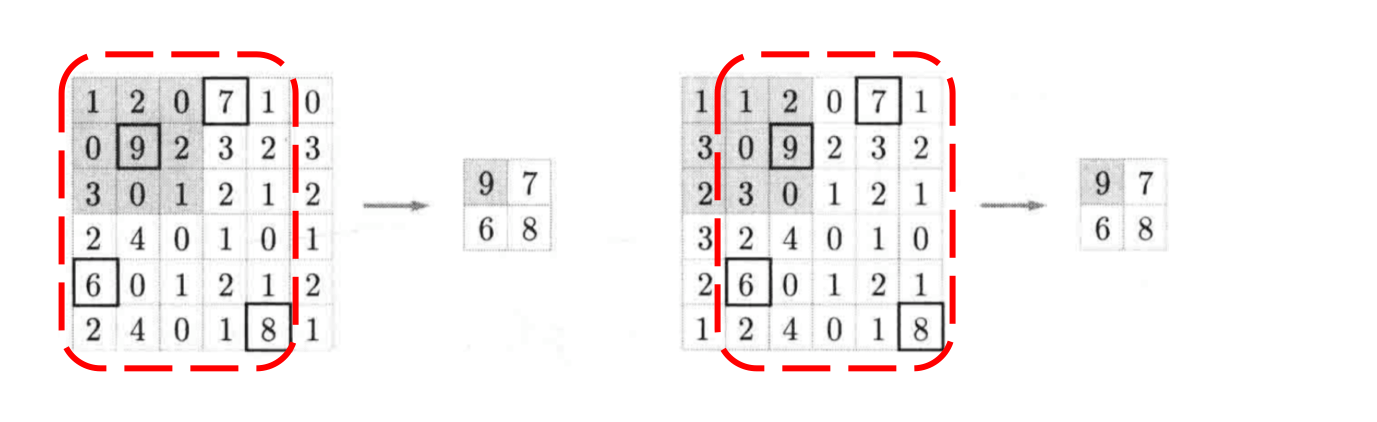- 由于池化之后特征图会变小，如果后面连接的是全连接层，能有效的减小神经元的个数，节省存储空间并提高计算效率

## 3. 应用示例

$$H_{out} = \frac{H + p_{h1} + p_{h2} - k_h}{s_h} + 1$$

$$W_{out} = \frac{W + p_{w1} + p_{w2} - k_w}{s_w} + 1$$

$$H_{out} = \frac{H}{2}$$

$$W_{out} = \frac{W}{2}$$

$$H_{out} = \frac{H + p_{h1} + p_{h2} - k_h}{s_h} + 1=\frac{4 + 0 + 0 - 2}{2} + 1=\frac{4}{2}=2$$

$$W_{out} = \frac{W + p_{w1} + p_{w2} - k_w}{s_w} + 1=\frac{4 + 0 + 0 - 2}{2} + 1=\frac{4}{2}=2$$

 - 池化窗口的初始位置为左上角，对应粉色区域，此时输出为 $3.5 = \frac{1 + 2 + 5 + 6}{4}$ ；

 - 由于步幅为2，所以池化窗口向右移动两个像素，对应蓝色区域，此时输出为 $5.5 = \frac{3 + 4 + 7 + 8}{4}$ ；

 - 遍历完第一行后，再从第三行开始遍历，对应绿色区域，此时输出为 $11.5 = \frac{9 + 10 + 13 + 14}{4}$ ；

 - 池化窗口向右移动两个像素，对应黄色区域，此时输出为 $13.5 = \frac{11 + 12 + 15 + 16}{4}$ 。

## 4. 反向梯度计算

CNN网络中另外一个不可导的环节就是Pooling池化操作，因为Pooling操作使得feature map的尺寸变化，假如做2×2的池化，假设那么第l+1层的feature map有16个梯度，那么第l层就会有64个梯度，这使得梯度无法对位的进行传播下去。其实解决这个问题的思想也很简单，就是把1个像素的梯度传递给4个像素，但是需要保证传递的loss（或者梯度）总和不变。根据这条原则，mean pooling和max pooling的反向传播也是不同的。

### 4.1. 平均池化

MeanPooling的前向传播就是把一个patch中的值求取平均来做pooling，那么反向传播的过程也就是把某个元素的梯度等分为n份分配给前一层，这样就保证池化前后的梯度（残差）之和保持不变，图示如：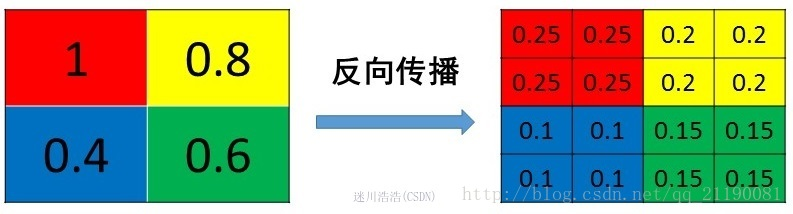MeanPooling比较容易让人理解错的地方就是会简单的认为直接把梯度复制N遍之后直接反向传播回去，但是这样会造成loss之和变为原来的N倍，网络是会产生梯度爆炸的。

### 4.2. 最大池化

MaxPooling也要满足梯度之和不变的原则，MaxPooling 的前向传播是把patch中最大的值传递给后一层，而其他像素的值直接被舍弃掉。那么反向传播也就是把梯度直接传给前一层某一个像素，而其他像素不接受梯度，也就是为0。所以MaxPooling操作和MeanPooling操作不同点在于需要记录下池化操作时到底哪个像素的值是最大，也就是max id，这个变量就是记录最大值所在位置的，因为在反向传播中要用到，那么假设前向传播和反向传播的过程就如下图所示 ：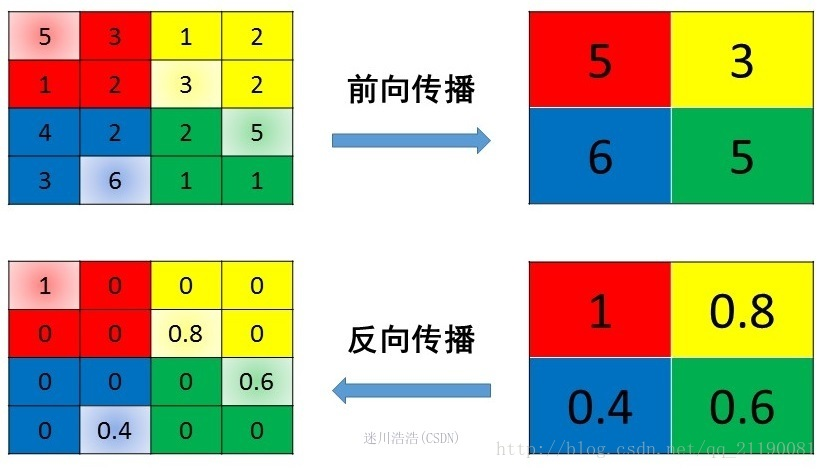Last modification：September 18th, 2021 at 11:01 am